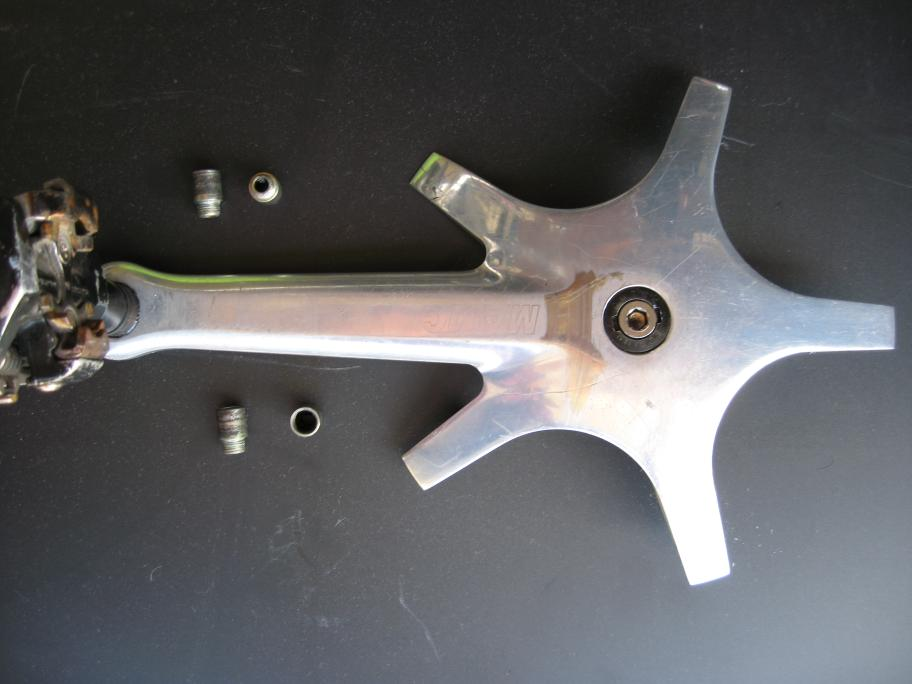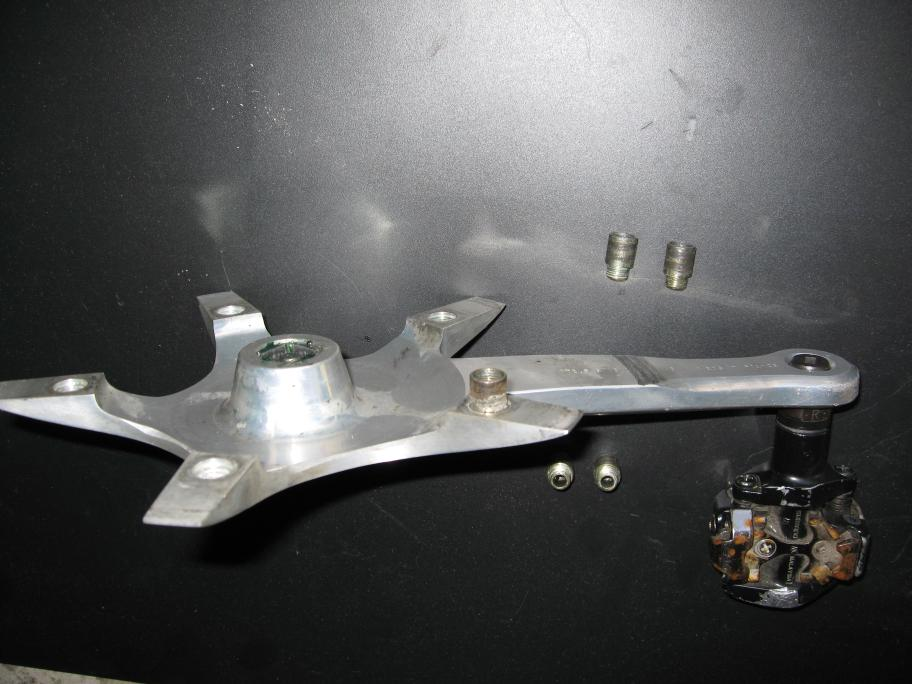MOBI > Mavic 631 "Starfish" Cranks

Mavic 631 "Starfish" cranks use a large thin spider outboard of all the chainrings. Unlike conventional cranks where the arm extends from pedal eye to crank spindle, the 631 becomes thin in the spider area.Even some light riders report chainring rub when pushing hard on the pedals. It is interesting to consider the torsional stiffness of the "starfish" spider against loads at the 3 o'clock position, compared to the stiffness of the arm with the pedal at the same position.

### Measurements and Calculations

As background, the torsional stiffness of a solid (not hollow) cylinder rises with the 4th power of the cylinder diameter, that is r4.

To a first/crude approximation, the torsional stiffness of a general beam is related to the stiffness of the largest "inscribed cylinder", that is the largest-diameter cylinder that fits within the cross-section of the beam. Stiffness is (roughly) proprotional to the stiffness of the largest inscribed cylinder multiplied by that ratio to the total beam cross section — that is, if the total area is 6 and the area of the cylinder is 2, then the total stiffness is 6/2=3 times the stiffness of the cylinder.

stiffness = (cylinder stiffness) × (total area)/(cylinder area)

The Mavic arm tapers slightly from the pedal eye to the "starfish", but is pretty close to 1/2" (sorry for the inch units, old measuring tools). The stiffness would be proportional to

0.54 = 0.0625

The area of the largest inscribed cylinder is

4/3 × π × (0.5/2)2 = 0.262 square inches

The arm is roughly 1.2" x 0.5" but the outer face is sloped, making the total cross section aree slightly less, perhaps

1.2 × 0.5 × 0.9 = 0.54 square inches

or about 2.1 times the area of the inscribed cylinder.

0.54 × 2.1 = 0.131 total section stiffness

The "starfish" tapers from the arm towards the spindle, getting thicker again near the spindle. The "starfish" is thickest on a line going from the pedal eye to the BB spindle. The thinnest part of that line is about 5mm before it starts thickening near the spindle. At that point, it is about 0.285" thick, so the largest inscribed cylinder has stiffness proportional to

0.2854 = 0.00659

The area of the largest inscribed cylinder is

4/3 × π × (0.285/2)2 = 0.0821 square inches

Consider a line through the "starfish" that is at right angles to the crank, roughly the slight line in the lower photo above. The thickness of the "starfish" varies along that line from 0.285" in the center to as little as 0.185" near the edge. The area is thus ROUGHLY

4.37 × 0.285 × 0.75 = 0.934 square inches

The area of the largest inscribed cylinder is 0.0821 square inches, so the section area is about 0.934/0.0821=11.4 times the section area of the largest inscribed cylinder. Thus, the total stiffness in the section would be proportional to

0.2854 × 11.4 = 0.0752 total section stiffness

Comparing the two section stiffnesses:

0.0752 / 0.131 = 5.75

Total pedal twisting under load depends on both the stiffness of the beam and the length of the beam. The flexy part of the "starfish" is only about 20mm, compared to a total length of about 170mm, so only about 10% is more flexy. Thus, if the flex of a full-length thick arm is 1.0×170, and if we take the flexiness of the "starfish" to be 5.0, then flex of the arm and starfish together is roughly

1.0 × (170 - 20) + 5.0 × 20 = 250